HOME plateWON | World!OfNumbers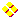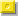Multiple Palindromic Primesof the type "Four in a row"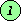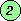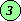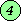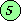bonham1Introduction

Here are some new observations about small palindromic primes. Russell Bonham named
them Multiple Palindrome Primes having a fixed set of properties further on explained
in mathematical detail.
As an introduction I would like to give some examples so that one knows immediately
what we are talking about. Let me begin with the general expression

N(p,q,k,m) = p.10(2k) + q.10(k+m) + q.10(k-m) + p

If we fill in k = 9, p = 1 in the first and last term we get 1.1018 + 1 which has a length of 19 digits
In order to arrive at palindromic primes the overall length must always be uneven, otherwise it
would always be divisible by 11.

[A] 10000000000000000001

Now for the two middleterms let us take q = 3, k is already known to be 9, we get another variable m to play with.

Let us take m = 2, we can finally construct the whole number

q.10(k+m) + q.10(k-m)
3.10(9+2) + 3.10(9-2)
3.1011 + 3.107
300000000000 + 30000000 = 300030000000

add this to [A] we finally have constructed our candidate to check for primeness

1000000300030000001

As you can detect there are always four digits spaced out by zeroes.
Hence the "Four in a row" in the title zone.

Attentive readers can see now that by playing around with the value of m we can space out the two
middle digits or shrink them in. Of course the two middle digits must stay within the boundaries
set up by the value of k.
Adding the other numbers with m=1, m = 3 up to m = 8 we get

1000000030300000001 → k=9, p=1, q=3 & m=1
1000000300030000001 → k=9, p=1, q=3 & m=2
1000003000003000001 → k=9, p=1, q=3 & m=3
1000030000000300001 → k=9, p=1, q=3 & m=4 [PRIME]
1000300000000030001 → k=9, p=1, q=3 & m=5
1003000000000003001 → k=9, p=1, q=3 & m=6
1030000000000000301 → k=9, p=1, q=3 & m=7
1300000000000000031 → k=9, p=1, q=3 & m=8

The pattern becomes obvious now. It is important to choose
correct values for p and q. For instance the combination p = 7 and q = 2
makes only numbers divisible by 3 and can thus never be prime.
Only 22 cases of p & q can cause primeness (see Table 1).
Note in the above example only one prime appears with value m = 4.
All the others are composite.

Russell Bonham investigated 'the number of primes' for larger and larger values of k (up to k=1500).

p000......0000000000q0q0000000000......000p
p000......000000000q000q000000000......000p
p000......00000000q00000q00000000......000p
p000......0000000q0000000q0000000......000p
p000......000000q000000000q000000......000p
p000......00000q00000000000q00000......000p
p000......0000q0000000000000q0000......000p
p000......000q000000000000000q000......000p

He poured his results and statistics in nice tables. Consult them further on and enjoy them!

Article by Russell Bonham ( email )

Multiple palindrome primes (PRPUs) with the same
sum over all integers, same number of digits and the
smallest number of non zero integers (4).

Palprimes involving one or two non zero integers of the form p0I0p or
p0I0q0I0p cannot possess multiple primes with the same number of
integers and the same sum over all integers with the possible exception
of 10^k + 1 where 11 and 101 are the only primes found so far. The simplest
example of palprimes with these properties are numbers of the form

N(p,q,k,m) = p.10(2k) + q.10(k+m) + q.10(k-m) + p

where m can take on all values from m = 1 to m = k – 1. This palindrome
number has the properties that its total number of digits is 2k+1 and the
sum over all digits is 2(p+q).

For numbers of this type the largest has the largest value of k. If two or
more numbers have the same value of k the largest number is the one with
the largest value of p. If numbers have the same value of both k and p the
size is determined by the largest value of m. In case all three constants are
the same the final size arbiter is the size of q.

In order to be prime N must have an odd number of digits, which it does, p
cannot be an even integer or 5, p + q cannot be divisible by 3, and p cannot
be equal to q. Earlier work suggests that p = q = 1 palindrome numbers
cannot be prime. This leaves 22 possible values of combinations of p and q
for the production of palprimes as shown in Table 1 where primes with fixed
p, q, and k with the largest values of k < 1501 and the most number of mUs
are given. Also given in Table 1 are the total number of primes found in a
search from k = 2 to 751 and a search from k = 752 to 1501 (3003 digits).

The total number of primes found for fixed values of p and q and a
particular range of k values is roughly constant (about 10% standard
deviation) as shown in Table 2. If the number of primes found is A for a
search over a range of k values dk where the average k value for the
range is k(ave) then the prime density, D, can be shown to be

D = A/{dk [ k(ave)+0.5]}

Hence the prime density falls off linearly with the average value of k for a
series of searches of constant width dk.

It is possible to have multiple primes with the same total number of digits
and sum over all digits. This corresponds to fixed values of p, q, and k and
a prime search over values of m from 1 to k-1. There are 8 different sets of
combinations of p and q as shown in Table 3. A second property of possible interest
is to fix the sum of p + q and value of k and look for the primes N(p,q,k,m) and
N(q,p,k,m) with the same value of m. Note that in the previous case p and q are not
always the same pair of integers. These two primes are identical in all respects
except that p and q in one prime becomes q and p in the other prime. Of course the
larger of the two primes is the one with the larger value of p. Table 4 shows the
possible five groups for k < 1501 where the sum of p and q is the same.

Table 1: Largest palprimes with k < 1501 with the largest number
of m values and the same values of k, p, and q.
Number of palprimes between k = 2 and 751 and between 752 and 1501
are displayed in the last two columns.
casepqkm# of primes
k = 2-751
# of primes
k= 752-1501
1131154119,132,223,775,827,1087507509
2141095420,502,670,952,956609595
316848132,167,695,713,732450456
417140835,314,483,610,929619641
51973079,124,289,447,469634574
63180815,48,113,603,640,783555550
7321488137,492,920,1346583572
83498495,123,139,262,758598529
93576031,68,133,272,334,508,575,650,658534515
1037118493,225,396,659,722478508
11381357158,718,1042,1210383366
12711282553,832,1151,1183,1223619652
13731362257,455,611,840,905,1236521543
14741396523,751,841,1107,1281422442
15761452494,623.634,811,1090,1437419468
167947234,45,92,327,370,446625625
17911350135,245,437,1180, 1231,1340549617
189278067,149,50310575
19941493610,1024,1316,1330490463
20951277576.864.1130,1210419423
21971090235,373,680,809,868632589
22981280482,775,1137,1154,1208491508

 k = 1000 → 1100 74 k = 1100 → 1200 67 k = 1200 → 1300 84 k = 1300 → 1400 83 k = 1400 → 1500 78 Average +/- std. dev. 77 +/- 7 k = 1500 → 1600 93 k = 1600 → 1700 74 k = 1700 → 1800 87 k = 1800 → 1900 72 k = 1900 → 2000 81 Average +/- std. dev. 81 +/- 9

Table 3. Largest palindrome primes with fewer than 1500 digits
with the same sum over all digits and the same number of digits
kpqmTotal
# digits
Sum of
digits
6551364,342,371,45813138
31334,408,434
51514105,217,232,299103310
3217,307,443
3231657,103,32364914
34152,193,272,286
124764,5025126
9414,96,118
4717934,45,92,327,370,44694532
9777,265,286,294,425
6871734,61137716
3534,200
71218,373,407,501,594
49838232,276,39699922
74146,236,326
92None
4531949,340,44090920
37108
73252,365,371,412,424
91193,401

Table 4: Palprime pairs N(p,q,k,m) and N(q,p,k,m).
The largest palprimes found are shown for all pairs k < 1501
and the total number found in each category except for the case
of 1 - 3 and 3 - 1 where the three largest primes are also listed.
Range of kp(1)q(1)p(2)q(2)kmNumber of pairs found
1-150013314122637
1-1500133111566887
1-1500133112323667
1-150017713362
1-150019916296224
1-150037731841734
1-1500799752291

Extra N(p,q,k,m) data research
Number N is prime for value(s) m

 N(1,3,9,m) → m = 4 N(1,4,9,m) → m = 2 N(1,6,9,m) → none N(1,7,9,m) → none N(1,9,9,m) → none N(3,1,9,m) → none N(3,2,9,m) → none N(3,4,9,m) → none N(3,5,9,m) → m = 2 N(3,7,9,m) → none N(3,8,9,m) → none N(7,1,9,m) → m = 2, 8 N(7,3,9,m) → none N(7,4,9,m) → m = 2 N(7,6,9,m) → none N(7,9,9,m) → none N(9,1,9,m) → none N(9,2,9,m) → none N(9,4,9,m) → m = 6 N(9,5,9,m) → m = 2, 8 N(9,7,9,m) → m = 4 N(9,8,9,m) → none

From the 22 * 8 or 176 candidates (with k = 9),
just 10 numbers turn out to be prime.

Can you find the smallest value k > 9 yielding no primes at all ?

```

```

[TOP OF PAGE]

Patrick De Geest - Belgium- Short Bio - Some Pictures